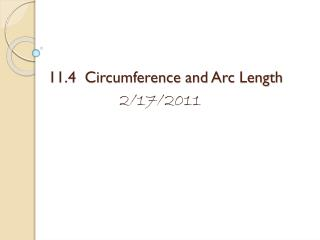Download Presentation11.4 Circumference and Arc Length

# 11.4 Circumference and Arc Length - PowerPoint PPT PresentationDownload Presentation## 11.4 Circumference and Arc Length

- - - - - - - - - - - - - - - - - - - - - - - - - - - E N D - - - - - - - - - - - - - - - - - - - - - - - - - - -
##### Presentation Transcript

1. 11.4 Circumference and Arc Length 2/17/2011

2. Objectives • Find the circumference of a circle and the length of a circular arc. • Use circumference and arc length to solve real-life problems.

3. Finding circumference and arc length • The circumference of a circle is the distance around the circle. • For all circles, the ratio of the circumference to the diameter is the same. This ratio is known as  or pi.

4. Theorem 11.6: Circumference of a Circle • The circumference C of a circle is C = d or C = 2r, where d is the diameter of the circle and r is the radius of the circle.

5. Ex. 1: Using circumference • Find (Round decimal answers to two decimal places) • (a) the circumference of a circle with radius 6 centimeters and • (b) the radius of a circle with circumference 31 meters.

6. What’s the difference?? • Find the exact radius of a circle with circumference 54 feet. • Find the radius of a circle with circumference 54 feet.

7. Extra Examples Write below previous box • Find the exact circumference of a circle with diameter of 15. • Find the exact radius of a circle with circumference of 25.

8. And . . . • An arc length is a portion of the circumference of a circle. • You can use the measure of an arc (in degrees) to find its length (in linear units).

9. Finding the measure of an Arc Length • In a circle, the ratio of the length of a given arc to the circumference is equal to the ratio of the measure of the arc to 360°. m • 2r Arc length of = 360°

10. More . . . ½ • 2r • The length of a semicircle is half the circumference, and the length of a 90° arc is one quarter of the circumference. d ¼ • 2r

11. Ex. 2: Finding Arc Lengths • Find the length of each arc. a. c. 50° 100°

12. Ex. 2: Finding Arc Lengths • Find the length of each arc. b. 50°

13. Ex. 2: Finding Arc Lengths • Find the length of each arc. c. 100°

14. Find the length of each arc. • 2(5) a. Arc length of = 50° 360° Ex. 2: Finding Arc Lengths # of ° a. • 2r a. Arc length of = 360° 50°  4.36 centimeters

15. Ex. 2: Finding Arc Lengths • Find the length of each arc. # of ° b. • 2r b. Arc length of = 360° 50° 50° • 2(7) b. Arc length of = 360°  6.11 centimeters

16. Find the length of each arc. Ex. 2: Finding Arc Lengths # of ° c. • 2r c. Arc length of = 360° 100° 100° • 2(7) c. Arc length of = 360°  12.22 centimeters In parts (a) and (b) in Example 2, note that the arcs have the same measure but different lengths because the circumferences of the circles are not equal.

17. Ex. 3: Tire Revolutions • The dimensions of a car tire are shown. • To the nearest foot, how far does the tire travel when it makes 8 revolutions?

18. Assignment p. 214 (1-10)